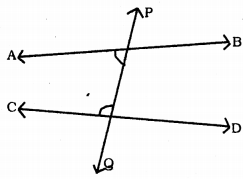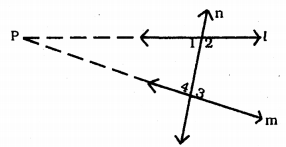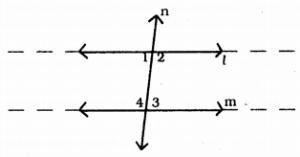# KSEEB Solutions for Class 9 Maths Chapter 2 Introduction to Euclid Geometry Ex 2.2

In this chapter, we provide KSEEB SSLC Class 9 Maths Chapter 2 Introduction to Euclid Geometry Ex 2.2 for English medium students, Which will very helpful for every student in their exams. Students can download the latest KSEEB SSLC Class 9 Maths Chapter 2 Introduction to Euclid Geometry Ex 2.2 pdf, free KSEEB SSLC Class 9 Maths Chapter 2 Introduction to Euclid Geometry Ex 2.2 pdf download. Now you will get step by step solution to each question.

## Karnataka Board Class 9 Maths Chapter 2 Introduction to Euclid Geometry Ex 2.2

Question 1.
How would you rewrite Euclid’s fifth postulate so that it would be easier to understand?
Euclid’s fifth postulate states that “If a straight line falling on two straight lines makes the interior angles on the same side of it taken together less than two right angles, then the two straight lines, if produced indefinitely meet on that side on which the sum of angles is less than two right angles.”For example, the line PQ falls on lines AB and CD such that the sum of the interior angles 1 and 2 is less than 180° on the left side of PQ. Therefore, the lines AB and CD will eventually intersect on the left side of PQ.
In the above figure, ∠APQ + ∠PQC < 180° then AB and CD meet at the side of A and C.

Question 2.
Does Euclid’s fifth postulate imply the existence of parallel lines ? Explain.‘n’ is a straight line which intersects l and m straight lines.
∠1 + ∠4 < 180° and ∠2 + ∠3 > 180°.
If l and m, produced by the side of ∠1 and ∠4 they meet at p.
If ∠1 + ∠4 < 180° and ∠2 + ∠3 > 180°,
then l and m straight lines do not meet even produced, which means they are parallel lines.

All Chapter KSEEB Solutions For Class 9 Maths

—————————————————————————–

All Subject KSEEB Solutions For Class 9

*************************************************

I think you got complete solutions for this chapter. If You have any queries regarding this chapter, please comment on the below section our subject teacher will answer you. We tried our best to give complete solutions so you got good marks in your exam.

If these solutions have helped you, you can also share kseebsolutionsfor.com to your friends.

Best of Luck!!